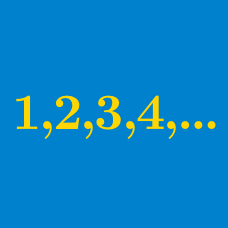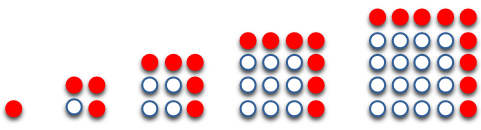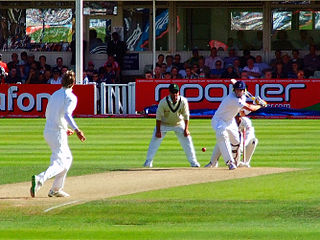Algebra

# Arithmetic Progression Sum

Evaluate

$1 + 3 + 5 + 7 + 9 + 11 + 13 + 15 + 17 + 19.$The number of red circles in the above diagram forms an arithmetic progression. What is the sum of the first 10 terms of this sequence?You're the captain of your cricket team which is taking part in a series of matches. In the first match you score 40 runs and in the last match you score 100 runs. Provided that your score increases by 12 runs in each match, find your total score throughout the series.

If the sum of first $n$ terms of an arithmetic progression is given by $S_n=3n^2+2n$, what is the 24th term of the AP?

$\begin{array} { l l l } 2 & = 2 & = 1 \times 2 \\ 2 + 4 & = 6 & = 2 \times 3 \\ 2 + 4 + 6 & = 12 & = 3 \times 4 \\ \vdots & \vdots & \vdots \\ \end{array}$

According to the above pattern, what is

$2 + 4 + 6 + \ldots + 30 ?$

×